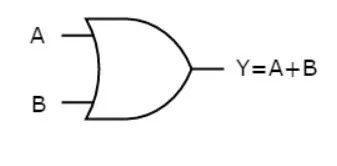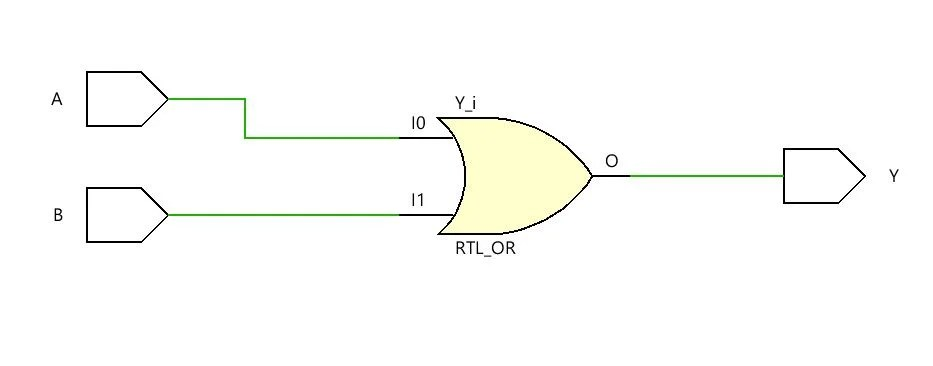View Course Path

# Verilog Code for OR Gate – All modeling styles

In this post, we will code the OR gate using three modeling styles available in Verilog: Gate Level, Dataflow, and Behavioral modeling. These are just modeling styles and do not affect the final hardware design that we are going to make. In every model, we get the same results, but abstraction levels and coding approaches are different. Let’s start with Gate Level modeling for the OR gate.

Contents

## Gate Level modeling

Designing a complex circuit using basic logic gates is the goal of gate-level modeling. We specify the gates of the circuit in our code. Verilog supports describing circuits using basic logic gates as predefined primitives. These primitives are instantiated like modules except that they are predefined in Verilog and do not need a module definition.

### Logic Circuit of the OR gate

The output of the OR gate is high if at least one of the inputs is high else the output is low. Here’s the logical representation of the OR gate.### Verilog code for OR gate using gate-level modeling

We can start writing the hardware description for the OR gate as follows:

`module OR_2(output Y, input A, B);`

We begin by declaring the module. `module`, a basic building block in Verilog HDL is a keyword here to declare the module’s name. OR_2 is the identifier. Identifiers are how we name the module. The list in parenthesis is the port list containing input and output ports. You can check out the many other ways in which you can declare the I/O ports in a Verilog program. Then we write:

```    or(Y, A, B);
endmodule```

Verilog has this functionality to describe the circuit at the gate level. Here `or` is the operation performed on A, B, to get output Y. `endmodule` terminates the module.

Here, you can look on the complete code:

```module OR_2_gate_level(output Y, input A, B);
or(Y, A, B);
endmodule```

## Data flow modeling

Compared to gate-level modeling, dataflow modeling is a higher level of abstraction. Dataflow modeling describes the circuits by their function rather than by their gate structure. That becomes handy because gate-level modeling becomes very complicated for large circuits because let’s face it, a digital circuit with a bunch of gates can seem quite daunting.

Hence, dataflow modeling is a very important way of implementing the design. We require the boolean logic equation and continuous assignment statements to build the designs. The continuous assignments are made using the keyword `assign`.

### Equation of the OR gate

The boolean equation of an OR gate is Y = A + B.

### Verilog code for OR gate using data-flow modeling

We would again start by declaring the module.

`module OR_2_data_flow (output Y, input A, B);`

Then we use assignment statements in data flow modeling. Using

```assign Y = A | B;
endmodule
```

Just like the `or` operation, the `|` logical operator performs the operation of the inputs we write. Then `endmodule` is used to terminate the module.

## Behavioral Modeling

Behavioral modeling is the highest level of abstraction in the Verilog HDL. Behavioral models in Verilog contain procedural assignment statements, which control the simulation and manipulate variables of the data types. This level of abstraction simulates the behavior of the circuits without specifying the details.

We are not concerned about the fact that whether the circuit is implemented, for example, using NAND gates or NOR gates design. We are only focussed on the behavior of the design. Let’s have a look at the truth table.

### Truth Table for OR gate

 A B Y(A or B) 0 0 0 0 1 1 1 0 1 1 1 1

#### Equation from the truth table

Simply by minimization, (or you may arrive by k-maps), we can state that:

Y = A + B or say Y = A or B.

### Verilog code for OR gate using behavioral modeling

Again, we begin by declaring module, setting up identifier as `OR_2_behavioral`, and the port list.

`module OR_2_behavioral (output reg Y, input A, B);`

In this case, the port list includes the output and input ports. When our level of abstraction is behavioral level, then we use reg datatype in the output ports. The `reg` data object holds its value from one procedural assignment statement to the next and means it holds its value over simulation data cycles. You can read more about the reg data type in this post on data types in Verilog.

```Then we write,

always @ (A or B) begin
.....
end```

Using the `always` statement, a procedural statement in Verilog, we run the program sequentially.

`(A, B)` is known as the sensitivity list or the trigger list. The sensitivity list includes all input signals used by the `always` block. It controls when the statements in the always block are to be evaluated.

`@` is a part of the syntax, used before the sensitivity list. In Verilog, `begin` embarks and  `end` concludes any block which contains more than one statement in it.

Note that the always statement `always @(Y, A)` could be written as `always @ *.` ` *` would mean that the code itself has to decide on the input signals of the sensitivity list. Now, we have,

```always @ (A or B) begin
if (A == 1'b0 & B == 1'b0) begin
Y = 1'b0;
end
else
Y = 1'b1;
end```

The condition for the OR gate is that if both the inputs are low, then the output is also low, else in every other condition that has to be high.

`if (A == 1'b0 & B == 1'b0)` states that if both A and B are 0, then Y has to be 0, else 1.

Here is the full code:

```module OR_2_behavioral (output reg Y, input A, B);
always @ (A or B) begin
if (A == 1'b0 & B == 1'b0) begin
Y = 1'b0;
end
else
Y = 1'b1;
end
endmodule```

## RTL schematic of OR gate## Testbench of the OR gate using Verilog

The file to be included and the name of the module changes, but the basic structure of the testbench remains the same in all the three modeling styles. Here’s a guide on writing testbenches in Verilog.

````include "OR_2_behavioral.v"
module OR_2_behavioral_tb;
reg A, B;
wire Y;
OR_2_behavioral Indtance0 (Y, A, B);
initial begin
A = 0; B = 0;
#1 A = 0; B = 1;
#1 A = 1; B = 0;
#1 A = 1; B = 1;
end
initial begin
\$monitor ("%t | A = %d| B = %d| Y = %d", \$time, A, B, Y);
\$dumpfile("dump.vcd");
\$dumpvars();
end
endmodule```

## Simulation WaveformCorrectly depicting that whenever any of the input is high, the output is also high else the output is low.

This site uses Akismet to reduce spam. Learn how your comment data is processed.

Top